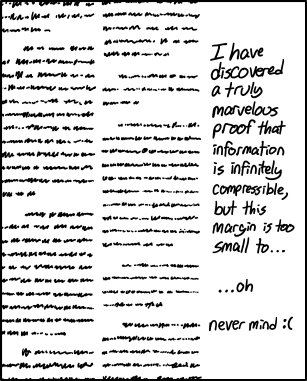## Fermat’s Riddle

Posted in Books, Kids, R with tags , , , , , , , , , , on October 16, 2020 by xi'an

·A Fermat-like riddle from the Riddler (with enough room to code on the margin)

An  arbitrary positive integer N is to be written as a difference of two distinct positive integers. What are the impossible cases and else can you provide a list of all distinct representations?

Since the problem amounts to finding a>b>0 such that$N=a^2-b^2=(a-b)(a+b)$

both (a+b) and (a-b) are products of some of the prime factors in the decomposition of N and both terms must have the same parity for the average a to be an integer. This eliminates decompositions with a single prime factor 2 (and N=1). For other cases, the following R code (which I could not deposit on tio.run because of the packages R.utils!) returns a list

```library(R.utils)
library(numbers)
bitz<-function(i,m) #int2bits
c(rev(as.binary(i)),rep(0,m))[1:m]
ridl=function(n){
a=primeFactors(n)
if((n==1)|(sum(a==2)==1)){
print("impossible")}else{
m=length(a);g=NULL
for(i in 1:2^m){
b=bitz(i,m)
if(((d<-prod(a[!!b]))%%2==(e<-prod(a[!b]))%%2)&(d<e))
g=rbind(g,c(k<-(e+d)/2,l<-(e-d)/2))}
return(g[!duplicated(g[,1]-g[,2]),])}}
```

For instance,

```> ridl(1456)
[,1] [,2]
[1,]  365  363
[2,]  184  180
[3,]   95   87
[4,]   59   45
[5,]   40   12
[6,]   41   15
```

Checking for the most prolific N, up to 10⁶, I found that N=6720=2⁶·3·5·7 produces 20 different decompositions. And that N=887,040=2⁸·3²·5·7·11 leads to 84 distinct differences of squares.

## editor’s nightmare

Posted in Books, Kids, pictures, University life with tags , , , , , on June 24, 2014 by xi'an